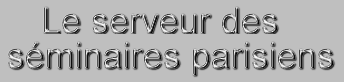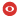Statut Confirmé Série IPN-THEO Domaines nucl-th Date Mercredi 26 Juin 2019 Heure 11:30 Institut IPN Salle Bâtiment 100, Salle A015 Nom de l'orateur Lynn Prenom de l'orateur Bryan W. Addresse email de l'orateur Institution de l'orateur Physics/CERCA/ISO, CWRU, Cleveland, OH, 44106 USA Titre Heavy nuclides as a liquid phase of $SU(2)_L \times SU(2)_R$ chiral perturbation theory: Emergence of semi-classical pion-less $SU(2) \chi$PT. Résumé Effective theories of nuclear structure must reflect the chiral global $SU(2)_L \times SU(2)_R$ symmetry of two-massless-quark QCD. Naive power counting enables perturbation/truncation in inverse powers of $\Lambda_{\chi^{SB}} \approx 1$GeV, with analytic operators renormalized to all loop orders. We show that $SU(2)~\chi$PT admits a liquid" phase, with energy required to increase or decrease the density of constituents. "Semi- classical Pion-less $SU(2)~\chi$PT emerges in the chiral liquid, vastly simplifying the derivation of saturated nuclear matter (the infinite liquid phase) and of finite microscopic liquid drops (ground-state heavy nuclides). Static Chiral Nucleon Liquids (Static $\chi$NL) are made entirely of nucleons, have even parity; total spin zero; even proton number $Z$, and neutron number $N$; and are arranged so local expectation values for spin and momenta vanish.\\ We derive the Static $\chi$NL effective Lagrangian, to order $\Lambda_{\chi^{SB}}$ and $\Lambda_{\chi^{SB}}^0$. Static $\chi$NL motivate nuclear matter, seen as non-topological solitons at zero internal and external pressure: the Nuclear Liquid Drop Model and Bethe-Weizsäcker Semi- Empirical Mass Formula emerge in an explicit Thomas-Fermi construction. For chosen nuclides, semi-classical nuclear Skyrme models are justified. We conjecture that inclusion of $\Lambda_{\chi^{SB}}^{-1}$ and $\Lambda_{\chi^{SB}}^{-2}$ operators will result in "natural" semi-classical Skyrme, No-Core-Shell, and non-exotic neutron star models, with approximate liquid structure. Numéro de preprint arXiv Commentaires  Fichiers attachés 2019_06_26_B_Lynn.pdf (232258 bytes)Pour obtenir l' affiche de ce séminaire : [ Postscript | PDF ]

 [ Annonces ]    [ Abonnements ]    [ Archive ]    [ Aide ]    [ JavaScript requis ] [ English version ]